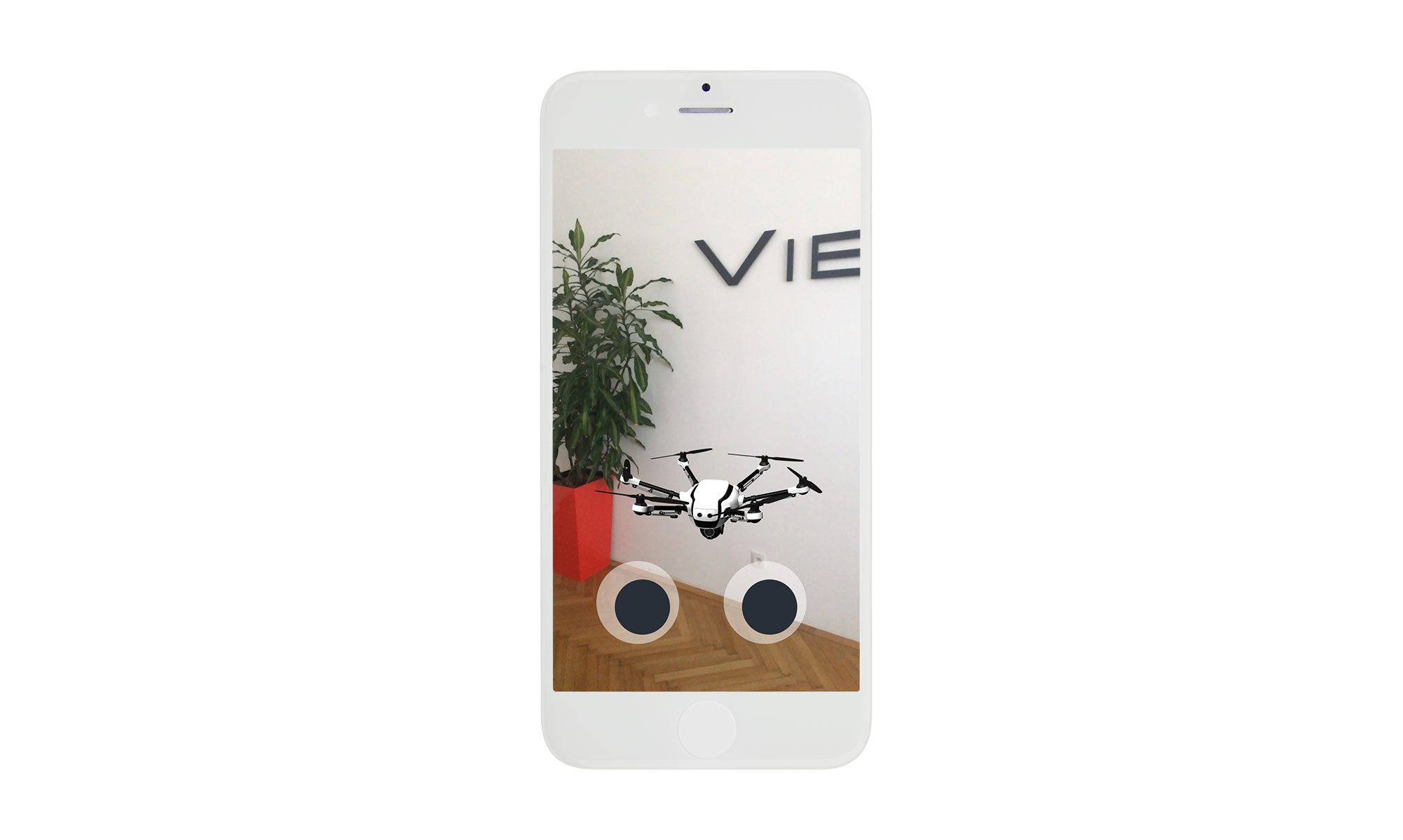Wouldn't be so much more fun, if the drone was flying in its look direction? Let's make it happen!

For that we need need to slightly edit the `handleRightJoystickInput` function. Start right before the pose of the model instance gets updated.

``````const handleLeftJoystickInput = ({ x, y }) => {
const pose = instance.pose;
{...}

// put your code right here!

instance.setPose(pose);
``````
##### Get the forward vector

First, we need to analyze the model instance to get its normal forward vector (Vector3.FORWARD).

``````const position = new Vector3(instance.pose.position);
``````

Now, we need to and apply the instance's orientation vector to it and adjust it to the speed of the move.

``````const forward = Vector3.FORWARD;
forward.rotate(newOrientation);
// alternatively: const forward = Vector3.FORWARD.rotate(newOrientation);
forward.scale(y * 20);  // You may also use a different value
``````
##### Update the position

Don't forget to apply your changes.

``````pose.position = position.add(forward);
``````

### Test it!

At this point, you should be able to manipulate the drone instance's position with the use of the joysticks.Screenshot from the Drone Control App

< Previous Step